Ex 8.1

Chapter 8 Class 7 Rational Numbers
Serial order wise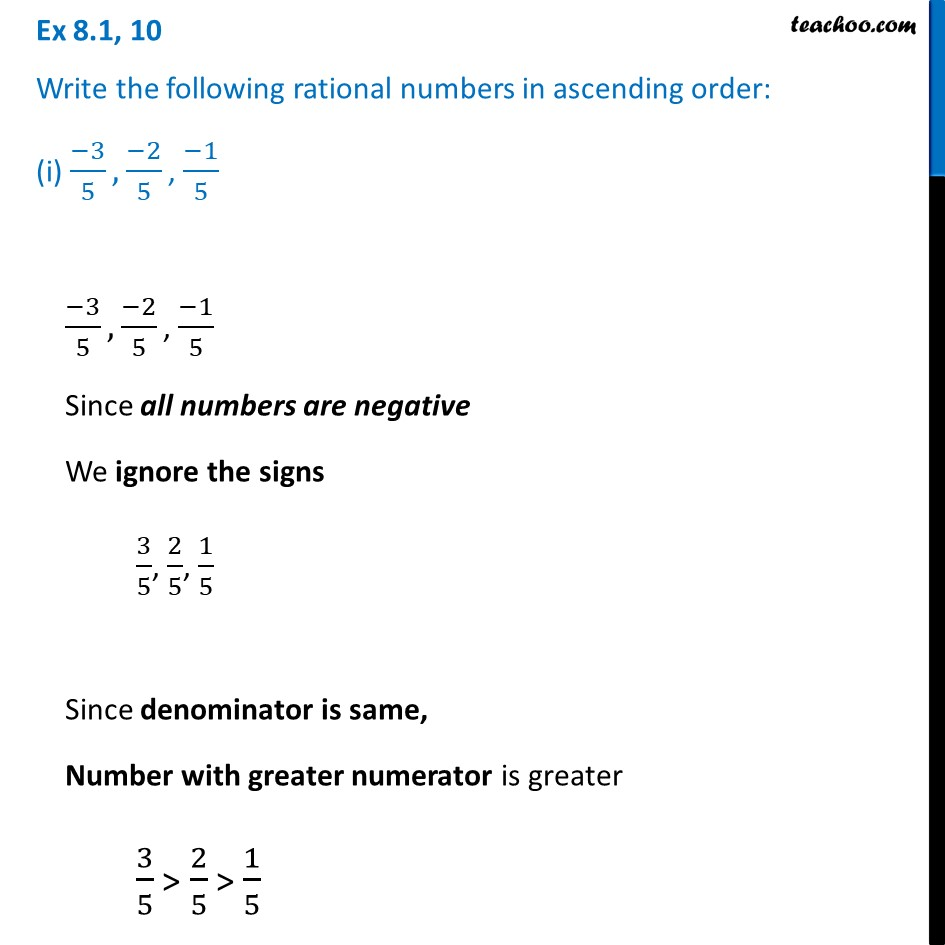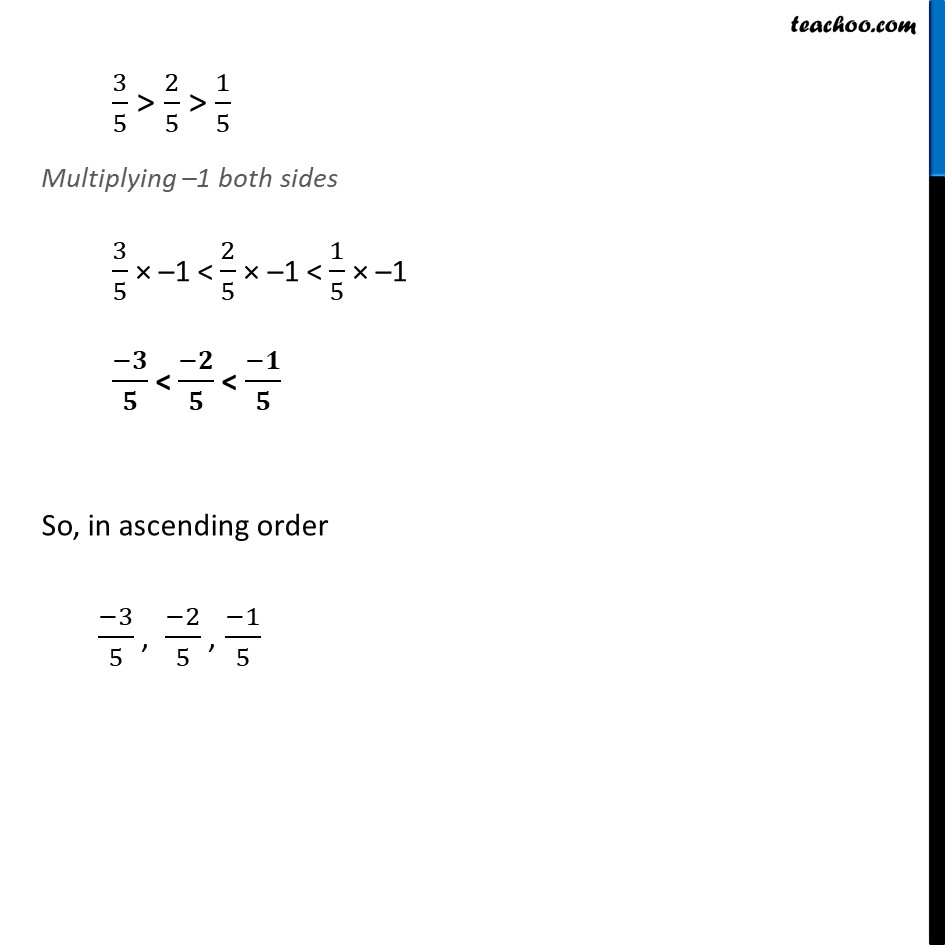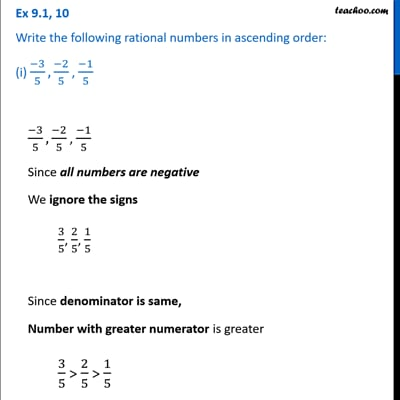This video is only available for Teachoo black users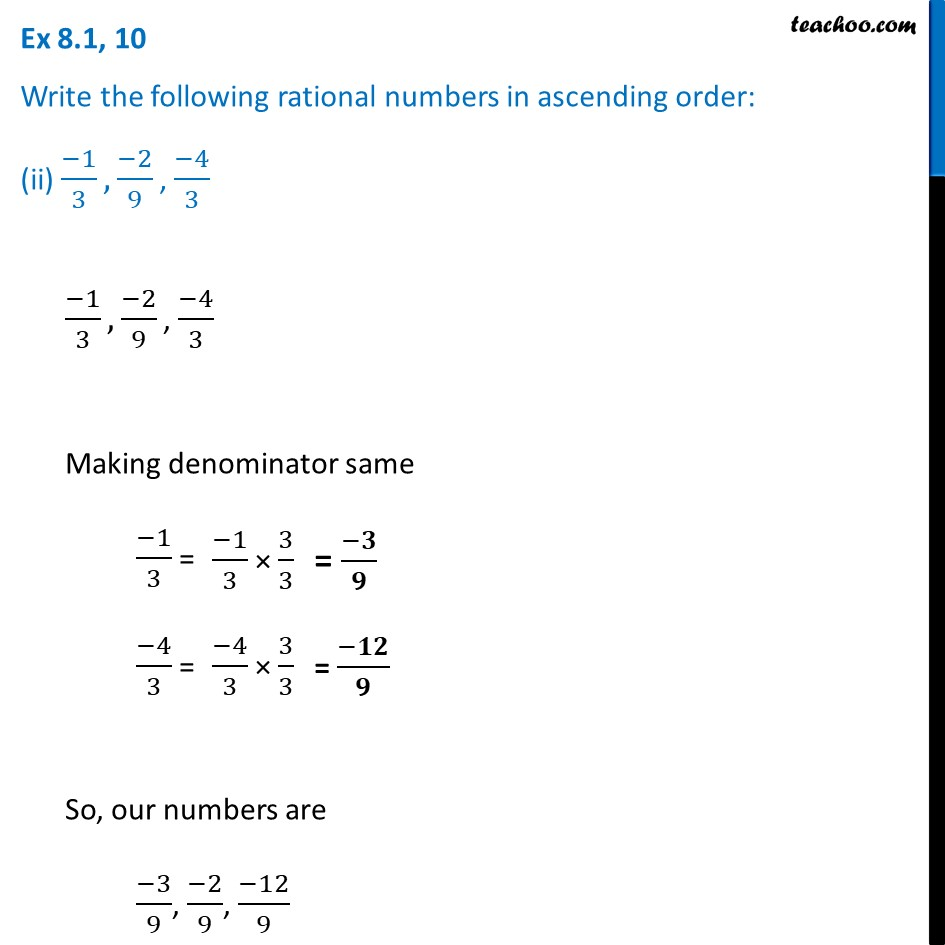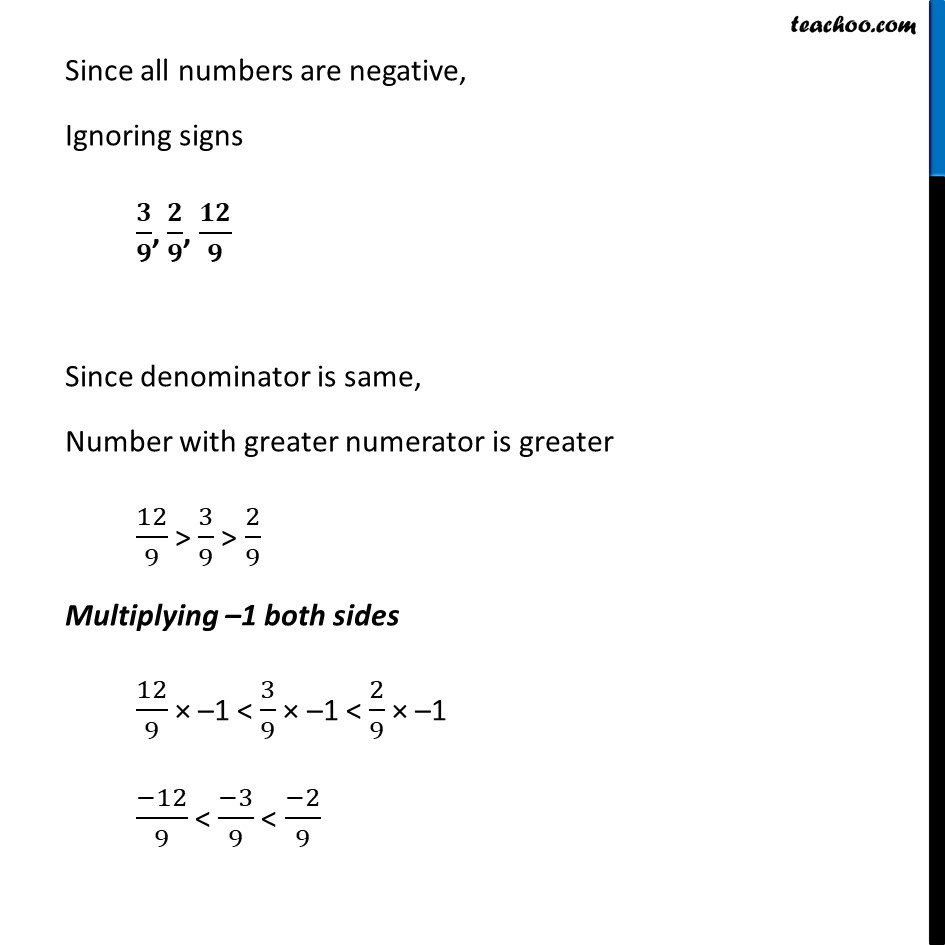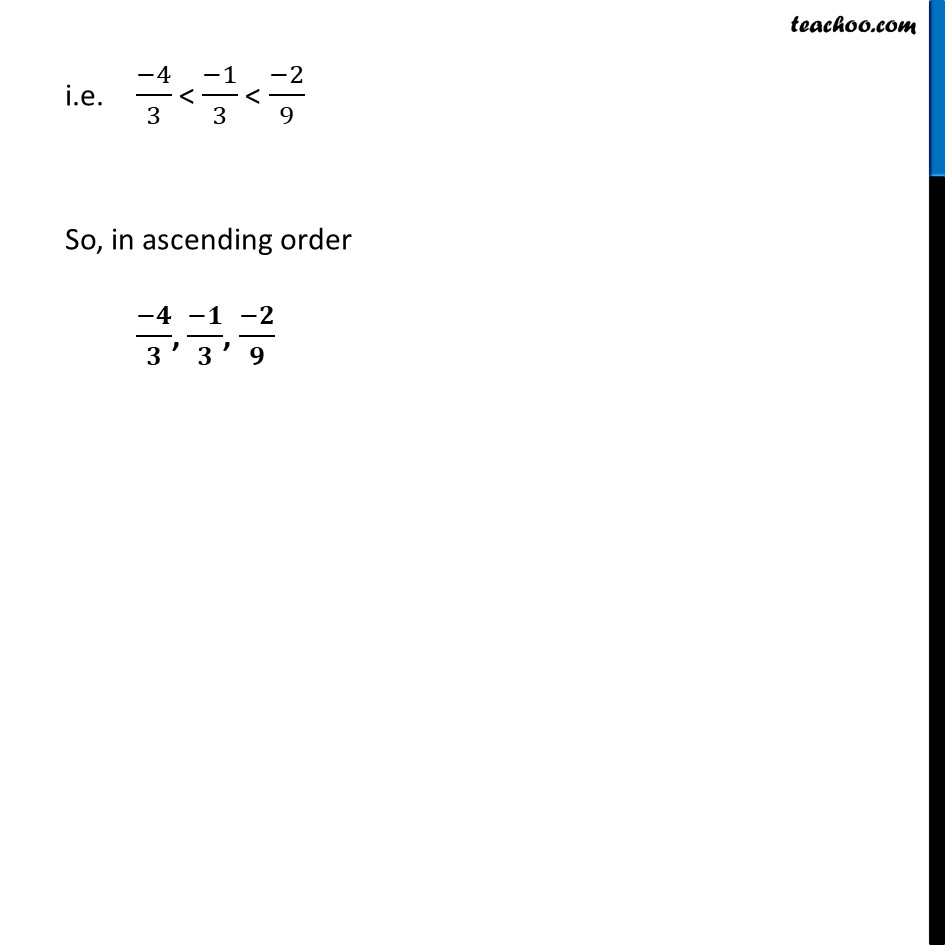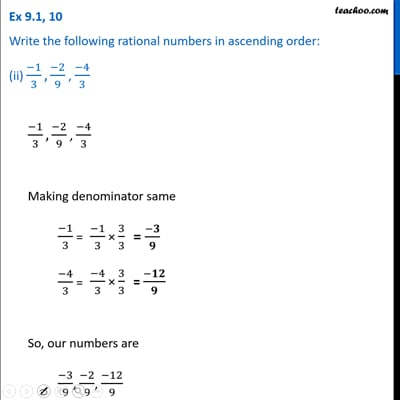This video is only available for Teachoo black users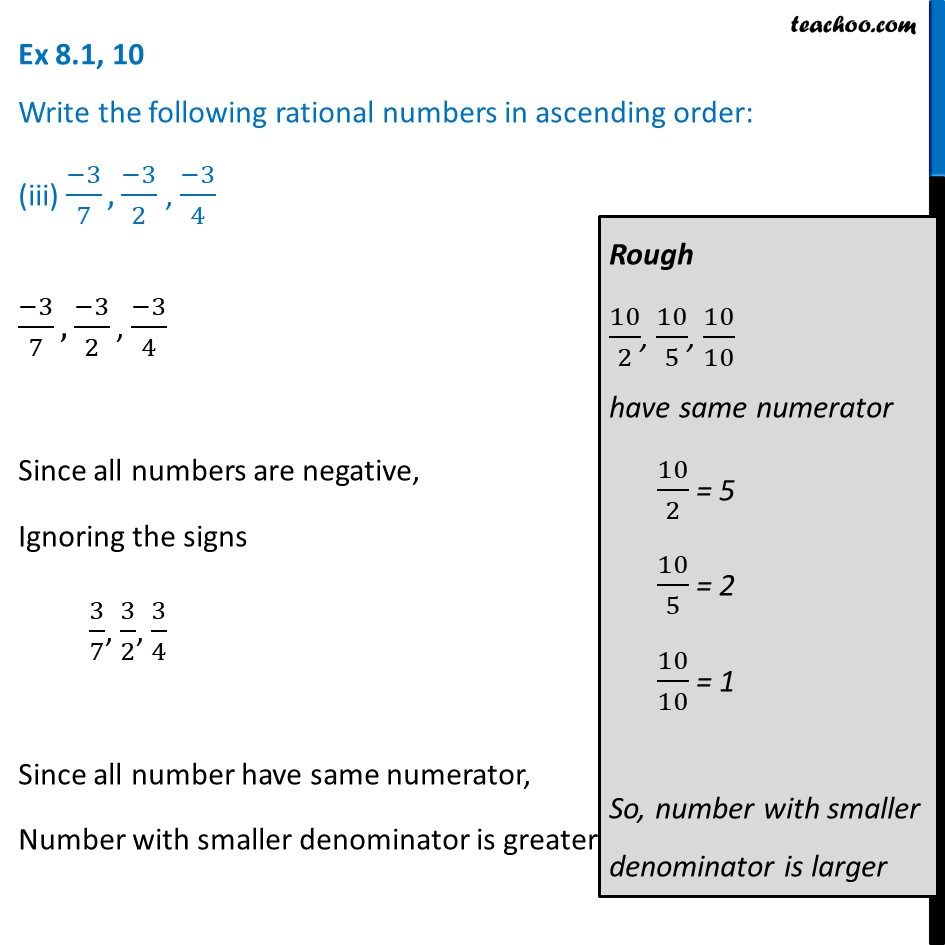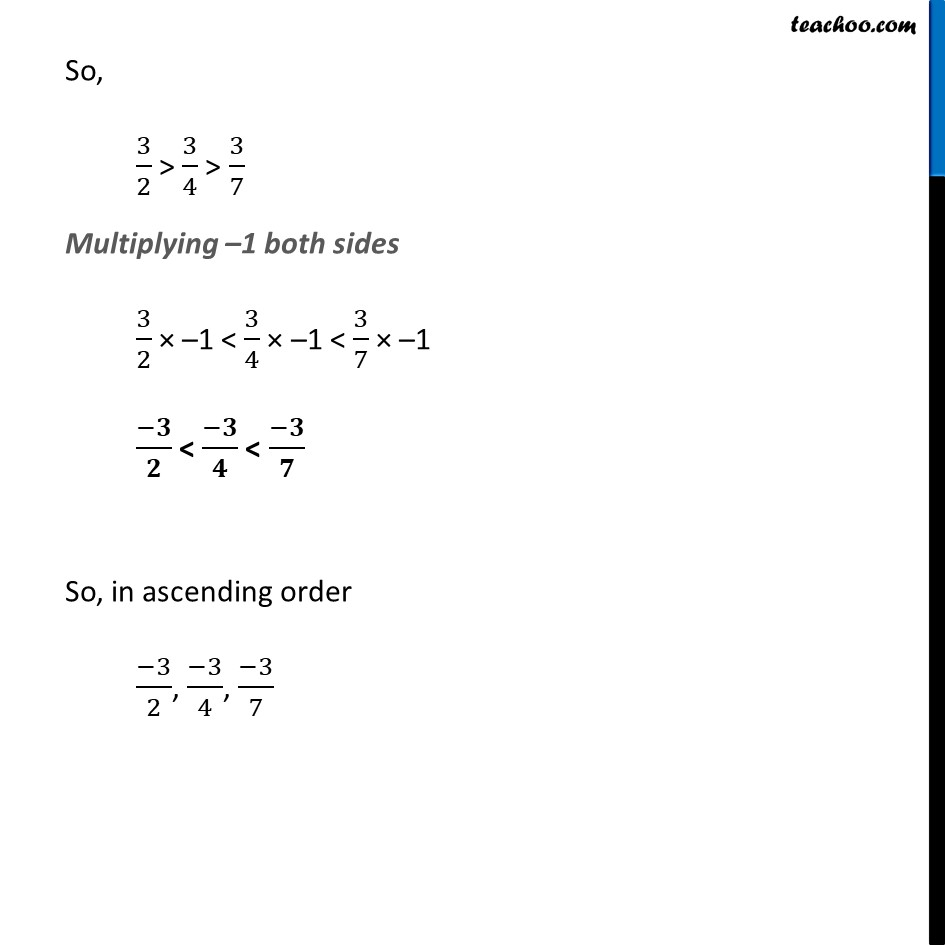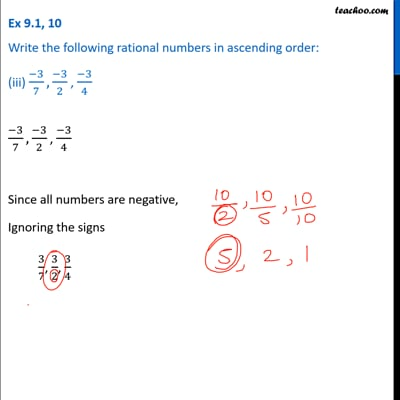This video is only available for Teachoo black users

Learn in your speed, with individual attention - Teachoo Maths 1-on-1 Class

### Transcript

Ex 8.1, 10 Write the following rational numbers in ascending order: (i) (−3)/5, (−2)/5 , (−1)/5 (−3)/5, (−2)/5 , (−1)/5 Since all numbers are negative We ignore the signs 3/5, 2/5, 1/5 Since denominator is same, Number with greater numerator is greater 3/5 > 2/5 > 1/5 3/5 > 2/5 > 1/5 Multiplying –1 both sides 3/5 × –1 < 2/5 × –1 < 1/5 × –1 (−𝟑)/𝟓 < (−𝟐)/𝟓 < (−𝟏)/𝟓 So, in ascending order Ex 8.1, 10 Write the following rational numbers in ascending order: (ii) (−1)/3, (−2)/9 , (−4)/3 (−1)/3, (−2)/9 , (−4)/3 Making denominator same (−1)/3 = (−4)/3 = So, our numbers are (−3)/9, (−2)/9, (−12)/9 Since all numbers are negative, Ignoring signs 𝟑/𝟗, 𝟐/𝟗, 𝟏𝟐/𝟗 Since denominator is same, Number with greater numerator is greater 12/9 > 3/9 > 2/9 Multiplying –1 both sides 12/9 × –1 < 3/9 × –1 < 2/9 × –1 (−12)/9 < (−3)/9 < (−2)/9 i.e. (−4)/3 < (−1)/3 < (−2)/9 So, in ascending order (−𝟒)/𝟑, (−𝟏)/𝟑, (−𝟐)/𝟗 Ex 8.1, 10 Write the following rational numbers in ascending order: (iii) (−3)/7, (−3)/2 , (−3)/4(−3)/7, (−3)/2 , (−3)/4 Since all numbers are negative, Ignoring the signs 3/7, 3/2, 3/4 Since all number have same numerator, Number with smaller denominator is greater Rough 10/2, 10/5, 10/10 have same numerator 10/2 = 5 10/5 = 2 10/10 = 1 So, number with smaller denominator is larger So, 3/2 > 3/4 > 3/7 Multiplying –1 both sides 3/2 × –1 < 3/4 × –1 < 3/7 × –1 (−𝟑)/𝟐 < (−𝟑)/𝟒 < (−𝟑)/𝟕 So, in ascending order (−3)/2, (−3)/4, (−3)/7